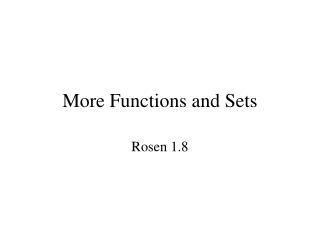DownloadDownload PresentationMore Functions and Sets

# More Functions and Sets

Télécharger la présentation## More Functions and Sets

- - - - - - - - - - - - - - - - - - - - - - - - - - - E N D - - - - - - - - - - - - - - - - - - - - - - - - - - -
##### Presentation Transcript

1. More Functions and Sets Rosen 1.8

2. B A S f(a) a Inverse Image • Let f be an invertible function from set A to set B. Let S be a subset of B. We define the inverse image of S to be the subset of A containing all pre-images of all elements of S. • f-1(S) = {aA | f(a) S}

3. B f f A -1 a2 b1 b2 a1 S f-1(S) Let f be an invertible function from A to B. Let S be a subset of B. Show that f-1(S) = f-1(S) What do we know? f must be 1-to-1 and onto

4. Let f be an invertible function from A to B. Let S be a subset of B. Show that f-1(S) = f-1(S) Proof: We must show that f-1(S)  f-1(S) and that f-1(S)  f-1(S) . Let x  f-1(S). Then xA and f(x)  S. Since f(x)  S, x  f-1(S). Therefore x  f-1(S). Now let x  f-1(S). Then x  f-1(S) which implies that f(x)  S. Therefore f(x)  S and x  f-1(S)

5. Let f be an invertible function from A to B. Let S be a subset of B. Show that f-1(S) = f-1(S) Proof: f-1(S) = {xA | f(x)  S} Set builder notation = {xA | f(x)  S} Def of Complement = f-1(S) Def of Complement

6. Floor and Ceiling Functions • The floor function assigns to the real number x the largest integer that is less than or equal to x. x • x = n iff n  x < n+1, nZ • x = n iff x-1 < n  x, nZ • The ceiling function assigns to the real number x the smallest integer that is greater than or equal to x. x • x = n iff n-1 < x  n, nZ • x = n iff x  n < x+1, nZ

7. Examples 0.5 = 1 0.5 = 0 -0.3 = 0 -0.3 = -1 6 = 6 6 = 6 -3.4 = -3 3.9 = 3

8. Prove that x+m = x + m when m is an integer. Proof: Assume that x = n, nZ. Therefore n  x < n+1. Next we add m to each term in the inequality to get n+m  x+m < n+m+1. Therefore x+m = n+m = x + m x = n iff n  x < n+1, nZ

9. n n+1 Let xR. Show that 2x = x + x+1/2 Proof: Let nZ such that x = n. Therefore n  x < n+1. We will look at the two cases: x  n + 1/2 and x < n + 1/2. Case 1: x  n + 1/2 Then 2n+1  2x < 2n+2, so 2x = 2n+1 Also n+1  x + 1/2 < n+2, so x + 1/2  = n+1 2x = 2n+1 = n + n+1 = x + x+1/2

10. Let xR. Show that 2x = x + x+1/2 Case 2: x < n + 1/2 Then 2n  2x < 2n+1, so 2x = 2n Also n  x + 1/2 < n+1, so x + 1/2  = n 2x = 2n = n + n = x + x+1/2

11. Characteristic Function Let S be a subset of a universal set U. The characteristic function fS of S is the function from U to {0,1}such that fS(x) = 1 if xS and fS(x) = 0 if xS. Example: Let U = Z and S = {2,4,6,8}. fS(4) = 1 fS(10) = 0

12. Let A and B be sets. Show that for all x, fAB(x) = fA(x)fB(x) Proof: fAB(x) must equal either 0 or 1. Suppose that fAB(x) = 1. Then x must be in the intersection of A and B. Since x AB, then xA and xB. Since xA, fA(x)=1 and since xB fB(x) = 1. Therefore fAB = fA(x)fB(x) = 1. If fAB(x) = 0. Then x  AB. Since x is not in the intersection of A and B, either xA or xB or x is not in either A or B. If xA, then fA(x)=0. If xB, then fB(x) = 0. In either case fAB = fA(x)fB(x) = 0.

13. Let A and B be sets. Show that for all x, fAB(x) = fA(x) + fB(x) - fA(x)fB(x) Proof: fAB(x) must equal either 0 or 1. Suppose that fAB(x) = 1. Then xA or xB or x is in both A and B. If x is in one set but not the other, then fA(x) + fB(x) - fA(x)fB(x)= 1+0+(1)(0) = 1. If x is in both A and B, then fA(x) + fB(x) - fA(x)fB(x) = 1+1 – (1)(1) = 1. If fAB(x) = 0. Then xA and xB. Then fA(x) + fB(x) - fA(x)fB(x)= 0 + 0 – (0)(0) = 0.

14. Let A and B be sets. Show that for all x, fAB(x) = fA(x) + fB(x) - fA(x)fB(x) A B AB fAB(x) fA(x) + fB(x) - fA(x)fB(x) 1 1 1 1 1+1-(1)(1) = 1 1 0 1 1 1+0-(1)(0) = 1 0 1 1 1 0+1-(0)(1) = 1 0 0 0 0 0+)-(0)(0) = 0